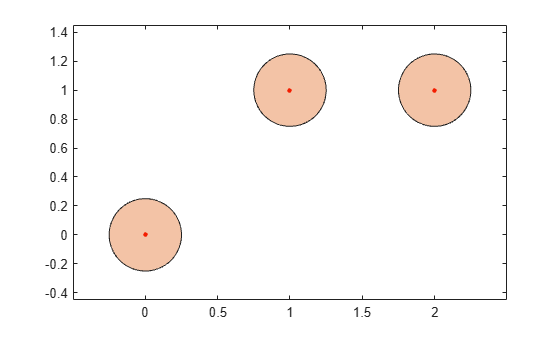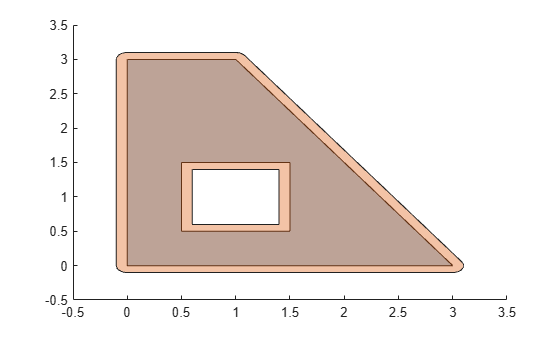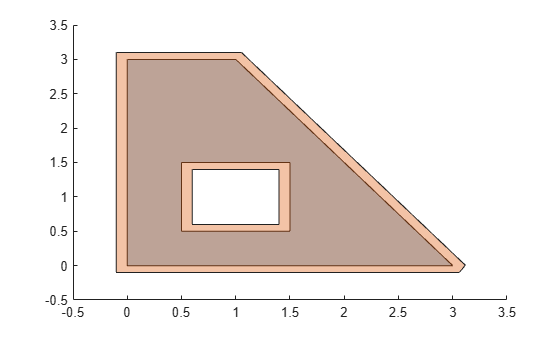# polybuffer

Create buffer around points, lines, or `polyshape` objects

## Syntax

``polyout = polybuffer(P,'points',d)``
``polyout = polybuffer(P,'lines',d)``
``polyout = polybuffer(P,'lines',d,'JointType',jt)``
``polyout = polybuffer(P,'lines',d,'JointType','miter','MiterLimit',lim)``
``polyout = polybuffer(polyin,d)``
``polyout = polybuffer(polyin,d,'JointType',jt)``
``polyout = polybuffer(polyin,d,'JointType','miter','MiterLimit',lim)``

## Description

example

````polyout = polybuffer(P,'points',d)` returns a `polyshape` object with boundaries that buffer the 2-D points in `P` by a distance `d`. The `polybuffer` function computes the buffer by taking the union of circles of radius `d` centered at each point in `P`. The first column of the matrix `P` contains the x-coordinates of the points to buffer and the second column contains the corresponding y-coordinates.Once you create the `polyshape` object `polyout`, you can analyze its properties or perform additional computations using `polyshape` functions. For example, you can access the vertices that define the buffered shape with the property `polyout.Vertices`, and you can plot the shape using the command `plot(polyout)`.```

example

````polyout = polybuffer(P,'lines',d)` returns a `polyshape` object with boundaries that buffer the line segments defined by the 2-D points in `P`. The `polybuffer` function computes the buffer by moving a circle of radius `d` along the line segments created by the input points, centered on the line segments.```

example

````polyout = polybuffer(P,'lines',d,'JointType',jt)` specifies how the meeting points of line segment endpoints (joints) are treated. For example, `polybuffer(P,'lines',d,'JointType','square')` squares off the joints.```
````polyout = polybuffer(P,'lines',d,'JointType','miter','MiterLimit',lim)` specifies a miter limit when the joint type is specified as `'miter'`. The miter limit is the maximum allowable ratio between the distance a joint vertex is moved and the buffer distance `d`. The limit `lim` must be greater than or equal to 2.```

example

````polyout = polybuffer(polyin,d)` returns a `polyshape` object with boundaries that buffer the input `polyshape` object `polyin` by a distance `d`. For positive values of `d`, solid region boundaries of `polyin` expand by `d` units, and hole boundaries shrink by `d` units. Negative values of `d` shrink solid boundaries and expand hole boundaries.```

example

````polyout = polybuffer(polyin,d,'JointType',jt)` specifies how the joints of the `polyshape` are treated when the first input argument is a `polyshape`.```
````polyout = polybuffer(polyin,d,'JointType','miter','MiterLimit',lim)` specifies a miter limit when the joint type is specified as `'miter'` and the first input argument is a `polyshape`.```

## Examples

collapse all

Create a matrix that contains the 2-D coordinates of three points. Then, create a `polyshape` object that buffers each point by a distance of 0.25 units.

```P = [0 0; 1 1; 2 1]; polyout = polybuffer(P,'points',0.25)```
```polyout = polyshape with properties: Vertices: [542x2 double] NumRegions: 3 NumHoles: 0 ```

Plot the original points and their buffer regions.

```plot(P(:,1),P(:,2),'r.','MarkerSize',10) hold on plot(polyout) axis equal```Create buffer regions surrounding line segments.

Create a matrix of 2-D points, and compute a `polyshape` object that buffers the line segments connecting the points.

```P = [0 0; 1 1; 2 1]; polyout1 = polybuffer(P,'lines',0.25); plot(P(:,1),P(:,2),'r.','MarkerSize',10) hold on plot(polyout1) axis equal hold off```By default, `polybuffer` rounds out the joints that connect line segments. You can control the shape of joints by using the `'JointType'` name-value pair. For example, use the value `'miter'` to preserve the angle connecting the two line segments.

```figure polyout2 = polybuffer(P,'lines',0.25,'JointType','miter'); plot(P(:,1),P(:,2),'r.','MarkerSize',10) hold on plot(polyout2) axis equal```Create a polygon with a solid boundary and a hole boundary. Then, create a buffer at a distance of 0.1 from the boundaries. By default the buffer has rounded joints.

```polyin = polyshape({[0 0 1 3],[0.5 1.5 1.5 0.5]},{[0 3 3 0],[0.5 0.5 1.5 1.5]}); plot(polyin) polyout1 = polybuffer(polyin,0.1); hold on plot(polyout1) hold off```Create a buffer using a miter limit of 2. The miter limit controls the pointiness of the joints.

```polyout2 = polybuffer(polyin,0.1,'JointType','miter','MiterLimit',2); plot(polyin) hold on plot(polyout2)```## Input Arguments

collapse all

Input vertices of 2-D points, specified as a 2-column numeric matrix. The first column of `P` contains the x-coordinates and the second column contains the corresponding y-coordinates.

Input `polyshape`, specified as a scalar, vector, matrix, or multidimensional array. When `polyin` is an array of `polyshape` objects, `polybuffer` applies the specified buffer parameters to each element.

Buffer distance, specified as a numeric scalar.

When the first input argument is a set of vertices, `d` must be a positive numeric scalar.

When the first input argument is a `polyshape`, `d` can be positive, negative, or zero:

• If `d>0`, then solid boundaries grow by a distance `d` and hole boundaries shrink.

• If `d<0`, then solid boundaries shrink by a distance `d` and hole boundaries grow.

• If `d=0`, then there is no change to the input boundaries.

Joint type for buffer boundaries, specified as one of the following:

• `'round'` — Round out boundary corners.

• `'square'` — Square off boundary corners.

• `'miter'` — Limit the ratio between the distance a joint vertex is moved and the buffer distance to 3. This limit prevents excessive pointiness.

When the first input argument is a set of vertices, `polybuffer` applies the joint type only where endpoints of two line segments meet, and not at an open endpoint of a line segment.

When the first input argument is a `polyshape`, `polybuffer` only applies the joint type to solid boundaries when the buffer distance is positive, or for hole boundaries when the buffer distance is negative.

Miter limit, specified as a positive numeric scalar greater than or equal to 2. The miter limit is the ratio between the distance a joint vertex is moved and the buffer distance. Setting a miter limit controls the pointiness of boundary joints.